## Microsoft® Excel 2013 Foundation

Certification Training
8809 Learners
5 Chapters +

# Apply Formulas and Functions Tutorial: Excel 2013 Foundation

This is the ‘Apply Excel Formula and Excel Functions’ tutorial of the Microsoft Excel 2013 MOS foundation course, offered by Simplilearn. In this tutorial, we will be learning various excel functions and excel formulas that we often come across while working with Excel.

## Objectives

By the end of this tutorial, we will be able to:

• Compare Excel formulas and Excel functions
• Describe cell references and ranges in formulas.
• Summarize data by using different functions.
• Use conditional logic in data summary functions
• Format and modify text by using text functions

## Formulas and Functions

A Formula is defined as an equation that performs calculations and returns the result value in cells. It contains several functions, cell references, operators on functions. A formula can be simple or complex based on users needs.

A Function can be defined as a built-in operation in Excel that returns a result value in cells. A function contains only cell references and values. Functions are tied alongside parenthesis were in the arguments, if any, are listed.

Formulas and Functions are similar, and they return results in cells. The only difference between a formula and function is that while formulas can be changed based on users needs, functions cannot.

### Cell References and Ranges in Formulas

A Cell Reference is defined as a cell or a cell range in a worksheet that can be used in a formula. This helps Excel too easily, find the data or values that need to be processed by a formula. There are three types of references in Excel 2013:

 Types Functions Relative Reference By default, each formula in Excel contains a relative reference. When we use a formula with a relative cell reference and copy the same formula into another cell, the formula reference changes. Usually, the relative reference will be identified as A1. If we want a formula cell reference to change automatically, we need to use relative cell reference. Absolute Reference or Fixed Reference If a cell reference is an absolute reference and formula is copied to another cell, the cell reference will not change. Usually, the absolute reference is identified as \$A\$1. If we want a formula to have a constant cell reference, we need to use a dollar sign before the column name and the Row number. Mixed Reference If we want to fix either a column or a row, then we use a mixed reference. We can fix a column or a row by adding a dollar sign before it. Mixed Reference is useful when a column needs to be fixed on a road needs to change, or when a road needs to be fixed or a column, needs to change. In other words, it can be described as a combination of absolute reference and a relative reference.

Using the correct type of cell reference in formulas ensures that they work as expected when copied to another location in the worksheet. Formulas and Functions are useful when using references. By default, cell addresses are dynamic in Excel 2013. It is possible to reference cells in other worksheets within a workbook or other workbooks.

Simplilearn has given a raise to all its employees. John’s manager has assigned him the task of calculating the revised net earnings for each employee after deducting taxes. He has asked John to add both the ‘tax amount’ and the ‘net earnings’ data to the earnings data sheet. John wants to do this using cell references in Excel 2013.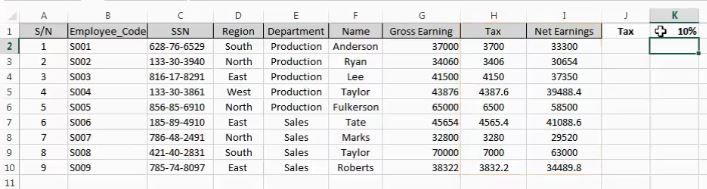Steps Performed in the above example:

• Select cells where results need to be displayed.
• Type “=” sign.
• Identify cell ranges to use in the formula.
• The Select cell containing the first value to be used in the formula.
• Add operator such as +, -, *, /.
• Select the cell containing the second value.
• Press Enter.
• The outcome is displayed in the results column.
• To apply the same formula to all the cells in the range, select the top-most cell displaying the result and double-click the fill(+) icon.

### Formula Calculation Order

Generally, every formula in mathematics uses the BODMAS rule. But in Excel 2013, we have the PEDMAS or BEDMAS rule.

### BODMAS

BODMAS checks for brackets, orders, power, square roots division, multiplication, addition, and subtraction in the given order.

### BEDMAS or PEDMAS

BEDMAS or PEDMAS checks for bracket or parenthesis, exponents, division, multiplication, addition, and subtraction in given order.

The only difference between BODMAS and BEDMAS is that while using BEDMAS in Excel, there are no orders on the formula. The calculation order is as below:

• Reference Operators(; : ,)
• Negation(-) such as -1.
• Percentage(%)
• Exponentiation(^)
• Division(/) and Multiplication(*)
• Concatenation(&) ampersand symbol to combine to cell values into single text
• Comparison(=, <>, <=, >=, <, >)

## SUM Function

In Excel 2013, the sum function allows us to add values in a data range or add specific cell values up to 255 cells.

Syntax: =SUM(Value1, value 2,......, value 255) or =Sum(select range of cells).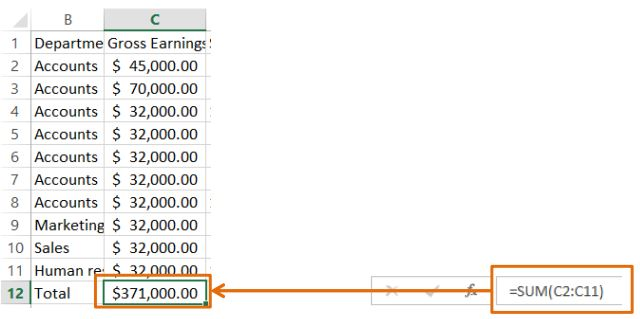The sum function is a built-in Excel function that ignores text values empty cells, and logical values. The shortcut key to writing the sum of a function for a data range is ALT+. When the sum function is used for a data range, any error values or arguments that are in text form are displayed as an error.

Simplilearn is about to start its annual financial review. John’s manager wants to know the total cost to the company from employee salary. John has the ‘individual employees’ gross earnings’ and wants to calculate the total using the SUM function in Excel 2013.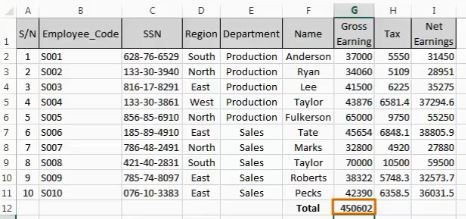Steps Performed in the above example:

• Select the cell where computed total should appear.
• Type =SUM( in the cell.
• Select the cell range has to be totaled.
• Enter.

## COUNT Function

In Excel 2013, the count function is used to count the number of cells containing numbers in a data range, or up to 255 cells. While using the count function Arguments, numbers, date or text representation of the numbers are counted.

Syntax: =COUNT(value1, value 2,......, value 255) or =COUNT(select range of cells).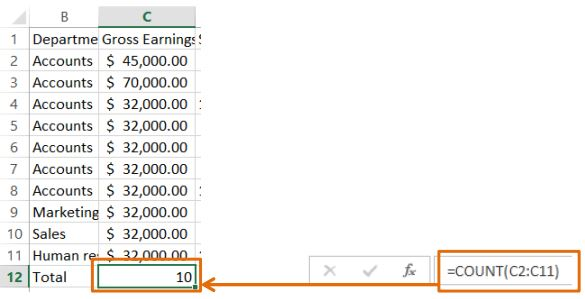Arguments that give error values or text, that cannot be translated into numbers are not counted.

## COUNTA Function

In Excel 2013, the COUNTA function is used to count the number of cells containing text, number and alphanumeric values in a given range, or up to 255 cells.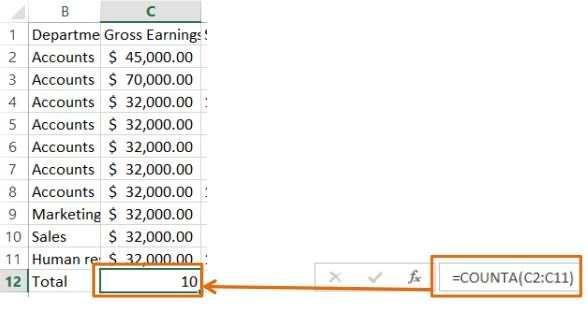Syntax: =COUNTA(Value1, value 2,......, value 255) or =COUNTA(select range of cells).

COUNTA function will count even if a cell contains space, but it gives logical and error values.

New employees have joined Simplilearn. John has added their data to the employee earnings sheet. John’s manager wants him to include the total number of salaried employees in the sheet. John wants to make use of the COUNT function in Excel 2013 to do this. John also notices that some employees haven’t been assigned their employee codes and wants to get a count of this using the COUNTA function.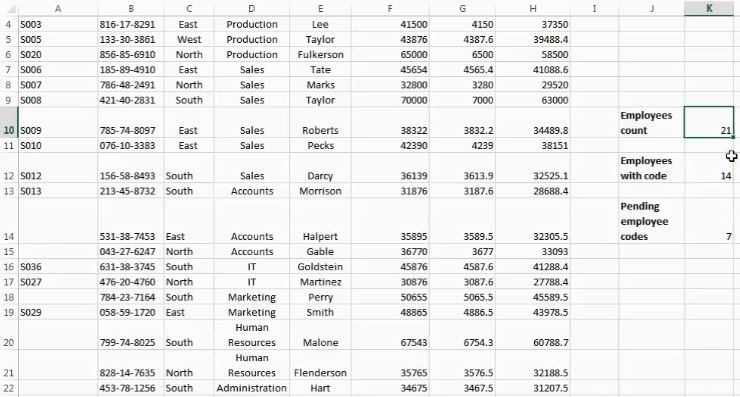Steps Performed in the above example:

• Identify the cell to display the number of entries in a range.
• For numeric values, select the cell and type ‘=count(‘.
• For non-numeric values, select the cell and type ‘=counta(‘.
• Press Enter
• If values are added to the cell range, select the cell displaying the count.
• Click the Error Checking Options button select the Update Formula to Include cells options.

## AVERAGE Function

The average function returns the average of a selected data range or up to 255 cells. The average function ignores text values, logical values, and empty cells.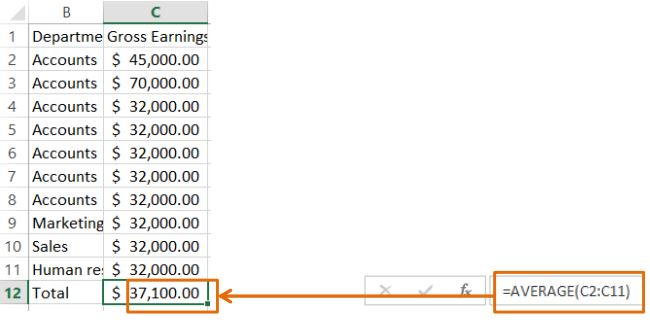Syntax: =AVERAGE(Value1, value 2,......, value 255) or =AVERAGE(select range of cells). Generally, the average function adds the number and then divides the result by the count of numbers.

## MIN Function

In Excel 2013, the MIN function returns the minimum (lowest) value in the data range or up to 255 cell values. The MIN function ignores empty cells, text values, and logical values.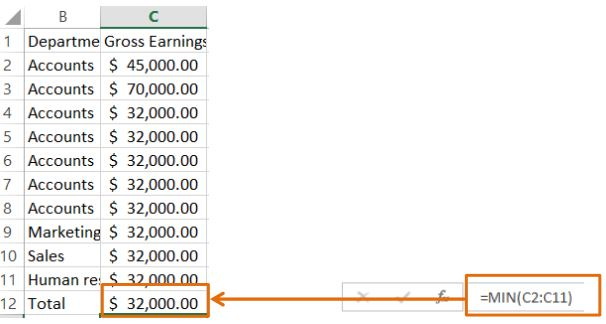Syntax: =MIN(Value1, value 2,......, value 255) or =MIN(select range of cells)

## MAX Function

In Excel 2013, the MAX function returns the maximum (highest) value in the data range or up to 255 cell values. The MAX function ignores empty cells, text values, and logical values.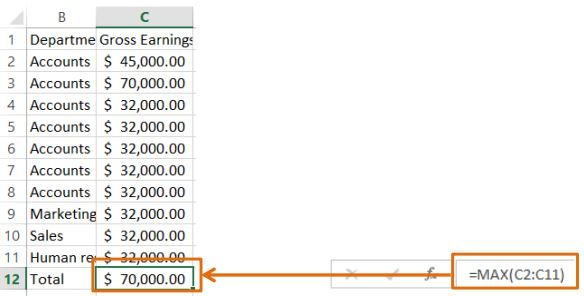Syntax: =MAX(Value1, value 2,......, value 255) or =MAX(select range of cells)

The HR manager requires the employee earnings data and has assigned John the task of providing the median earnings and the minimum and maximum earnings of employees. John wants to use the AVERAGE, MINIMUM, AND MAXIMUM functions in Excel 2013 to complete this task.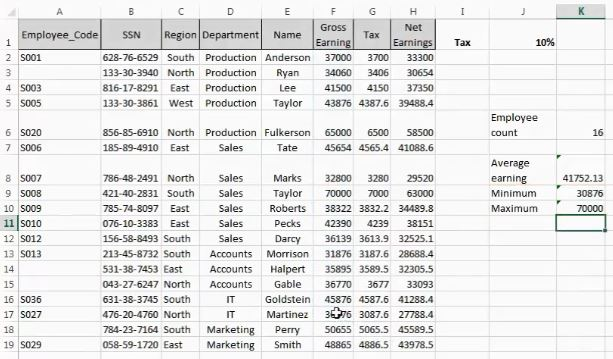Steps Performed in the above example:

• Identify the cell range on which to carry out the Average Minimum or Maximum functions.
• Identify the cells to display the average, minimum, or maximum values.
• Select the cell and type in the required Syntax: =average(, =min(, or =max(.
• Select the cell range on which the functions have to be performed.
• Press Enter.

## CONDITIONAL LOGIC

Conditional logic is generally used in data summary functions.

• Examples include SUMIF, SUMIFS, COUNTIF, COUNTIFS, AVERAGEIF, and AVERAGEIFS.
• The data summary functions will return a result only if a given condition is true.
• The comparison operators, such as Equal sign (=), Not equal sign (<>), Less than (<), Greater than (>), Less than or equal to (<=), and Greater than or equal to (>=), are used to create conditional logic.
• If we are working with conditional logic functions, an entire column needs to be selected whenever any new data is added so that the formula result is updated automatically

## SUMIF Function

The SUMIF function is used to sum a data range by using a criterion condition. For example, if we want to sum the earnings of a department, we can use the SUMIF function. The following arguments are required in the SUMIF function.

• Criteria Range: Select an entire column or only the desired data range as the Criteria Range. Criteria: Select a cell value or directly type the value. We can also use comparison operators.
• Sum_Range: Select an entire column or only the design data range, but usually we need to select values.

Syntax:  =SUMIF(criteria range, criteria, sum range)## SUMIFS function

The SUMIFS function is used to sum a data range by using multiple criteria. For example, if we want to add the earnings of a department, region wise, we can use the SUMIFS function. This function will sum the cell values only if the conditions are met. The following arguments are used in the SUMIFS function.

• Sum_Range: In SUMIF we need to select the sum range as the last argument, but in SUMIFS, we need to select the sum range first. Like SUMIF, we can add up to 127 criteria in SUMIFS.
• Criteria_range1:  Select criteria range, and it can be an entire column or only a few data cells.
• Criteria1: Select a cell value as criteria or type the value using comparison operators, along with wildcard characters Asterisk, question mark, and other symbols.

Syntax: =SUMIFS(sum range, criteria range1, criteria1, [criteria range2], [criteria2],……, [criteria range127], [criteria127])

Simplilearn is conducting its annual financial review. John’s manager has asked him to provide the department-wise expenditure on employee salaries and also the region-wise break-up of employee salaries for the Sales department. John wants to calculate the department-wise expenditure using the SUMIF function in Excel 2013. For including the region-wise employee earnings of the Sales department, he wants to use the SUMIFS function.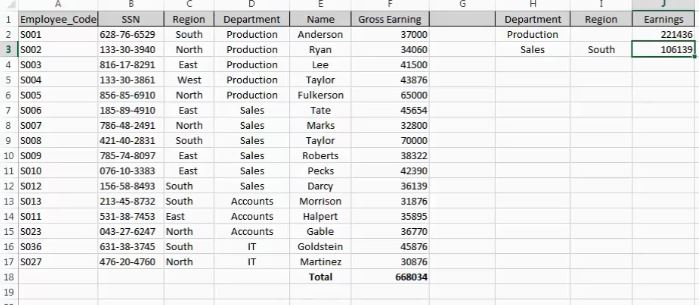Steps Performed in the above example:

• Identify the sum range and criteria to carry out the SUMIF and SUMIFS functions.
• Identify the cells to display the SUMIF or SUMIFS value.
• Select the cell and type in =sumif( or =sumifs( and press TAB.
• Click the Insert Function item under the Formulas tab.
• In the INSERT Function pop-up window, select the sum_range, criteria_range, and criteria to complete the argument for the given task.
• Click OK

## COUNTIF Function

The COUNTIF function is used to count the cells that contained data in a certain data range by using a criterion. For example, if we want to count the number of rows or records that contained the earnings of a department, we can use the COUNTIF function. The following arguments are used in COUNTIF function.

• Criteria Range: Select an entire column or only a specific data range as the criteria range.
• Criteria: Select a cell value or directly type the value. Comparison operators can be used.

Syntax: COUNTIF(criteria range, criteria)

## COUNTIFS Function

The COUNTIFS function is used to count the number of cells that contain the values of a data range by using multiple criteria conditions. For example, if we want to count the rows or records of earnings of a department region wise, we can use the COUNTIFS function. This function counts cells if all the conditions are met and also allows us to select up to 127 criteria.

The following arguments are used in the COUNTIFS function:

• Criteria Range1: Select criteria range from an entire column or only a specific data range.
• Criteria1: Select cell values as criteria or type the value using comparison operators.

We can use wildcard characters like an asterisk, question mark, and the tilde symbol.

Syntax:  =COUNTIFS(criteria range1, criteria1, [criteria range2], [c,riteria2],…….., [criteria range127], [criteria127])

Simplilearn is conducting its annual financial review. John has the employee earnings data for the Production and the Sales (South Region) departments. John’s manager has asked him to include the number of employees in each category in the same data sheet. John wants to use the COUNTIF and COUNTIFS functions for completing this task.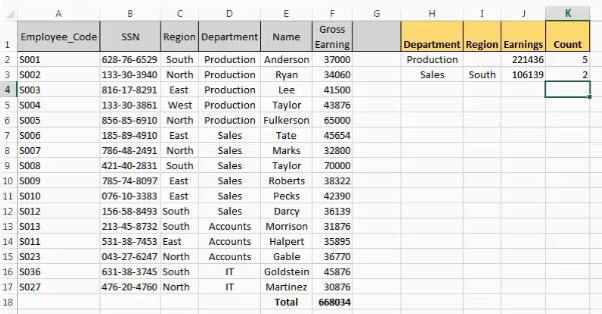Steps Performed in the above example:

• Identify the Criteria range and criteria to carry out the COUNTIF and COUNTIFS functions.
• Identify the cells to display the COUNTIF or COUNTIFS value.
• Select the cell and type in =countif( or countifs( and press TAB.
• Click the Insert Function item under the Formulas tab.
• In the INSERT Function pop-up window, select the sum_range, criteria_range, and criteria to complete the argument for the given task.
• Click OK

## AVERAGEIF Function

The AVERAGEIF function is used to get the average of a data range by using a criterion condition. For example, if we want to return the average earnings of a department, we can use the AVERAGEIF function. The following arguments are used in the AVERAGEIF function.

• Criteria Range: Select an entire column or only a specific data range as the criteria range.
• Criteria: Select a cell value or directly type the value. Comparison operators can also be used. Average Range: Select an entire column or only a specific data range as the average range. Usually we need to send values.

Syntax:  =AVERAGEIF(criteria range, criteria, average range)

## AVERAGEIFS Function

The AVERAGEIFS function is used to find the average of a data range by using multiple criteria. For example, if we want to return the average of the earnings of a department region wise, we can use the AVERAGEIFS function and this function will return the average of the cell values if all conditions are met. We can select up to 127 criteria.

The following arguments are used in the AVERAGEIFS function.

Average Range: In AVERAGEIF, the average range is selected as the last argument, but in AVERAGEIFS it is selected first because, in the AVERAGEIFS function, up to 127 criteria can be added.

• Criteria Range 1: An entire column, or only a specific data range is selected as the criteria range.
• Criteria1: Select a cell value as criteria or type the cell values using comparison operators. We can also use wildcard characters like an asterisk, question mark, and the tilde symbol.

Syntax:  =AVERAGEIFS(average range, criteria range1, criteria1, [criteria range2], [criteria2],…….., [criteria range127], [criteria127])

The HR at Simplilearn is planning to revise the pay scales of its employees. The HR manager has asked John to provide the department-wise average earnings data. She has also asked for a region-wise average of employee earnings for the Sales department. John wants to explore the AVERAGEIF and AVERAGEIFS functions in Excel 2013.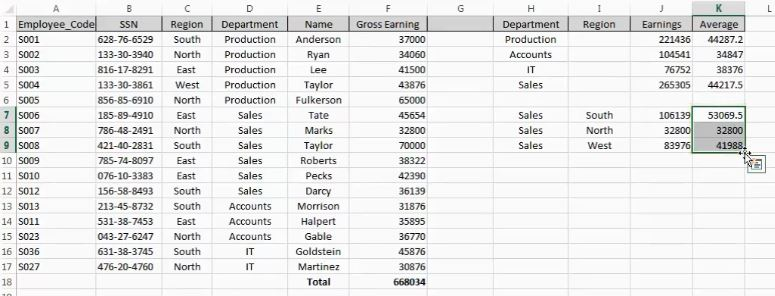Steps Performed in the above example:

• Identify the average range, criteria range and criteria to carry out the AVERAGEIF and AVERAGEIFS functions.
• Identify the cells to display the AVERAGEIF or AVERAGEIFS value.
• Select the cell and type in =averageif( or averageifs( and press TAB.
• In the INSERT Function pop-up window, select the average_range, criteria_range, and criteria to complete the argument for the given task.
• Click OK

## LOWER, UPPER, PROPER and CONCATENATE Functions

In Excel 2013, there are different text functions to format a modified data based on users needs.

Some basic data functions are:

• LOWER(text): Allows us to convert the text characters within a selected cell to lowercase letters.
• PROPER(text):  This function allows us to change the first character of each word within a cell to uppercase characters. Generally, this function is useful for titles.
• UPPER(text): This function allows us to change all text characters within a selected cell to uppercase letters.
• CONCATENATION: This function allows us to combine more than 2 cell values into a single value. Up to 255 cell values can be selected.

Syntax: =CONCATENATE(text1, text2, ……, text255)

While using CONCATENATE function, we can insert space or any symbol between two words or just using the symbol with double quotes. To CONCATENATE two or more strings use the ampersand(&) symbol between two words.

Excited to explore Excel formulas and Excel functions in detail. Get the course preview here!

## TRIM Function

When there is extra or unwanted space between words in our data, we can use the TRIM function to remove them. Only one argument is required for this function.

• Text: Select a cell value as the text argument.

Syntax: =TRIM(text)

Simplilearn is planning to organize a Sports Week for its employees and has asked the interested people to fill in their details on an Excel sheet. The text entries in the participant list are in different cases. John has been asked to edit the entries and remove the extra spaces. For this, he wants to use the LOWER, UPPER, CONCATENATE, PROPER, and TRIM functions.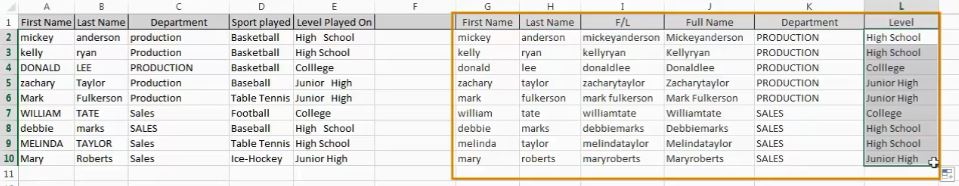Steps Performed in the above example:

• Identify the cells whose entries have to be edited.
• Select the cells to display the edited entries.
• To change the case to upper or lower, use the UPPER(type =upper() and LOWER(type =lower() functions.
• To concatenate text entries into two cells, use the CONCATENATE function(=concatenate(text1, text2, ….)
• To convert the text to the initial-capped format, use the PROPER function(=PROPER()
• To remove extra spaces in a cell, use the TRIM function(=trim()

## LEFT Function

When we need to divide a part of a text from the beginning of the text, we can use the LEFT function. It needs two function argument.

• Text: It is the text string from which the substring needs to be extracted.
• The Number of Characters: This argument refers to the number of characters that are required in the substring. Space is also considered. One space is equal to one character. If no numbers are entered, the function will consider one character by default.

Syntax:  =LEFT(text, number of characters)

The left function is generally used to get the first name from a full name or to derive the user id from an email id without a domain.

## RIGHT Function

The RIGHT function is used to divide a part of a text from the end of the text. It needs two function arguments.

• Text: This argument refers to the text string from which the substring needs to be extracted. It could also contain a reference to a column containing the required text.
• The Number of Characters: This argument refers to the number of characters required in the substring. Space is also considered. One space equals one character. If no numbers are entered, then the function considers it as one character by default.

Syntax: =RIGHT(text, number of characters)

The Right function is used to get the last name from a full name.

## MID Function

The Mid function is used to extract a string of characters from the middle of the text string that can start at any position in Excel 2013. Following arguments are required in the MID function:

• Text: It is the cell value from which the result needs to be extracted.
• Start number: This is the number of the middle word position.
• The Number of characters: Type the number of characters required in the extracted result.

Syntax:  =MID(text, start number, number of characters)

Simplilearn is planning to reorganize its branches and change the employee code format being used. John’s manager has assigned him the task of extracting the branch numbers from the employee code of each employee and the last four digits of their account numbers. John also has to indicate the employees’ departments with initials, such as P for Production. John wants to use the LEFT, RIGHT, and MID functions in Excel 2013 to do this.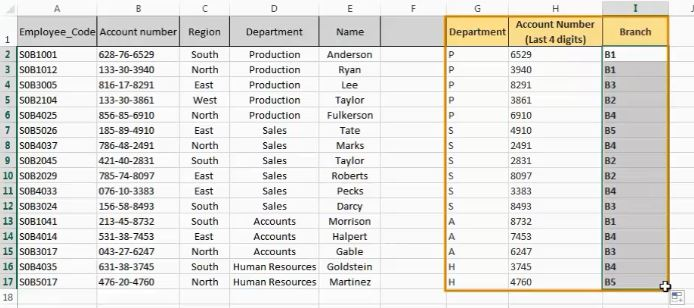Steps Performed in the above example:

• Identify the cells from where values are to be extracted.
• Select the cells to display the extracted values.
• To extract the values from the left, use the LEFT function(=LEFT(), select the target cell and specify the number of value to be returned. Click the arrow button next to column header.
• Similarly, to extract values from the right, use the RIGHT Function(=right().
• To extract value from the middle of an entry, type =MID(.
• Select the target cell, specify the starting number as well as the number of values to be returned.

## Key Takeaways:

Let us summarize what we've learned in this lesson.

• A formula is an equation that performs calculations, whereas a function is an Excel built-in operation.
• A reference is made in a formula to the content of a worksheet cell. It is of three types namely Relative reference, Absolute reference and Mixed reference.
• Excel 2013 uses Bedmas or Pedmas rule for formula calculations order.
• Functions, such as SUM, COUNT, AVERAGE, MIN, MAX, etc are used for summarizing data.
• Functions such as SUMIF, COUNTIF, AVERAGEIF, etc are used for performing conditional logic operations.
• Functions such as PROPER, TRIM, LEFT, RIGHT, etc are used to format and modify text.

## Conclusion

This concludes ‘Apply Functions and Formulas” tutorial.

Related Courses
Learner Reviews
Related Articles
• Disclaimer
• PMP, PMI, PMBOK, CAPM, PgMP, PfMP, ACP, PBA, RMP, SP, and OPM3 are registered marks of the Project Management Institute, Inc.

Name*
Email*
Phone Number*